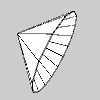# Py5Vector.rotate_around()#

Rotate around an arbitrary 3D vector.

## Examples#def setup():
py5.size(100, 100, py5.P3D)
py5.translate(10, 30, -20)
v1 = py5.Py5Vector(70, 0, -10)
cone_center = py5.Py5Vector(20, 10, 7)
with py5.begin_shape(py5.TRIANGLE_FAN):
py5.vertex(0, 0, 0)
for i in range(16):
v1.rotate_around(-py5.TWO_PI / 15, cone_center)
py5.vertex(v1.x, v1.y, v1.z)


## Description#

Rotate around an arbitrary 3D vector. This method is only applicable to 3D vectors. Use the angle parameter to specify the rotation angle and the v parameter to specify the vector to rotate around. The v vector does not need to be aligned to any axis or normalized, but it must be a 3D vector and it cannot be a vector of zeros.

The vector’s rotation will follow the right-hand rule. Using your right hand, point your thumb in the direction of the vector to rotate around. Your fingers will curl in the direction of rotation when the angle parameter is positive.

## Signatures#

rotate_around(
angle: float,  # angle of rotation, measured in radians
v: Py5Vector3D,  # 3D vector to rotate vector around
) -> Py5Vector3D


Updated on March 06, 2023 02:49:26am UTC